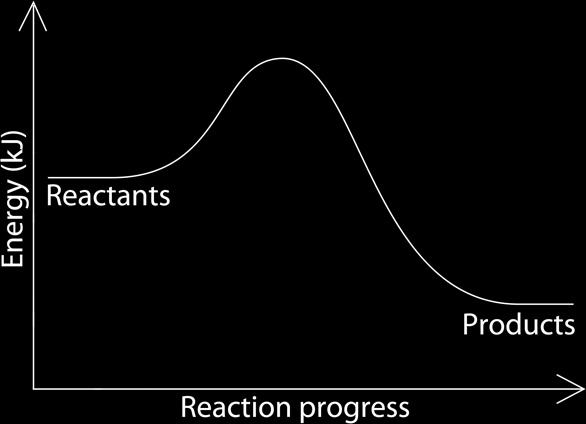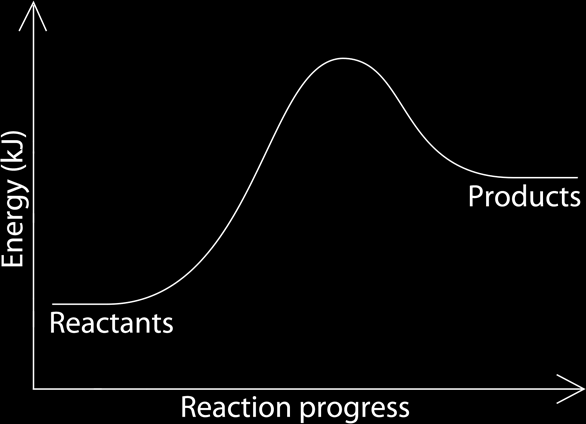# Energy in Chemistry

During any chemical reaction, an energy change occurs. Reactions release heat energy, or absorb heat energy from the surroundings. The ones that release heat energy to the surroundings are called Exothermic Reactions; the ones that absorb energy from the surroundings are called Endothermic Reactions. In exothermic reactions, the reactants are higher in energy than the products, in endothermic reactions; the reactants are lower in energy than the products.

### What makes a reaction exothermic or endothermic:

To start a reaction, a certain amount of energy is given to the reactants; this is called the Energy of activation because it activates the reaction. This energy is used to break the bonds between the atoms or molecules of the reactants, then the reactants rearrange and bond again, this bond formation releases energy. If the energy given to activate the reaction is less than the energy released during the bond formation, then the reaction gave out more energy than it took, so it is exothermic. If the energy given to activate the reaction is more than the energy released during bond formation, then the reaction took more energy than it gave out, so it is endothermic. The total energy change is called the enthalpy change or simply energy changed (ΔH). When the bonds are broken, the reaction is endothermic the ΔH value is positive, when bonds are formed, it is exothermic and the ΔH value is negative, the overall enthalpy change is:

Energy in - Energy out = ΔH

Endothermic reactions always have a positive ΔH and exothermic reactions always have a negative ΔH. Energy is measured in joules (J) or Kilojoules (KJ).

### Reaction and Energy Graph:

The energy change can be represented by a graph, the energy is on the y axis and the reaction progress is on the x axis. Endothermic and exothermic reactions have different graphs.

#### Exothermic Reactions:

In exothermic reactions, the reactants have more energy then the products, that is why small amounts of energy is required to activate the, and that is what makes is exothermic. The reactants having more energy than the products, make the amount of energy at the beginning of the reaction higher than at the end, the energy in between is given off creating a curve in the graph.#### Endothermic Reactions:

It is the opposite here, in endothermic reactions, the reactants have lower energy in them than the products, this makes them less stable, needing more energy to activate the reactions, that is why they are endothermic. Because of that, the beginning of the reaction has less energy than at end of it; the difference of amount of values is caused by absorbing energy from the surroundings.The overall energy change is determined by the amount of energy needed to activate the reaction and break the bonds and the amount of energy released during bond formation. So to calculate the overall energy change we have to know the amount of energy needed to break the bonds between the reactants and the amount of energy released during bond formation.

Calculate the enthalpy change when methane (CH4) reacts with oxygen (O2), given the following information.

CH4 + 2O2 → CO2 + 2H2O

 Bond Bong Energy (KJ/Mol) C-H 435 O=O 497 C=O 803 H-O 464 C-C 347 C-O 358

Energy needed to break bonds:

4 (C-H): 435 x 4 = 1740 KJ/Mol
2 (O=O): 497 x 1 = 994 KJ/Mol
Total energy in: 1740 + 994 = 2734 KJ/Mol

Energy needed to form bonds:
2 (C=O): 803 x 2 = 1606 KJ/Mol
4 (H-O): 464 x 4 = 1856 KJ/Mol
Total energy out: 1606 + 1856 = 3462 KJ/Mol

ΔH= 2734 – 3462 = -728 KJ/Mol r
Therefore reaction is Exothermic

When the ΔH is negative, the reaction is exothermic because the negative means that the reactants LOST energy to the surroundings. If it was positive the reaction is endothermic because the positive means that the reactants GAINED or ABSORBED energy from the surroundings.

#### Examples of Exothermic Reactions:

##### Combustion:

This is the reaction of any carbon containing fuel with oxygen producing carbon dioxide if complete combustion and carbon monoxide if incomplete combustion and large amounts of energy.

CH4 + 2O2 → CO2 + 2H2O + Energy

##### Respiration:

Burning food (glucose) in living organisms to produce energy and carbon dioxide.

C6H12O6 + 6O2 → 6CO2 + 6H2O + Energy

##### Hydration:

Adding water to salt powder, it is not dissolving. The powder changes to crystals.

CuSO4 + 5H2O → CuSO4.5H2O + Energy

##### Displacement:

A more reactive metal displaces the less reactive one.

Zn + CuSO4 → ZnSO4 + Cu + Energy

##### Neutralization:

Adding and acid to an alkali forming salt, water and energy.

HCl + NaOH → NaCl + H2O

#### Examples of Endothermic Reactions:

##### Photosynthesis:

Using carbon dioxide and water to make glucose and oxygen in the presence of light energy and chlorophyll.

6CO2 + 6H2O → C6H12O6 + 6O2

##### Dehydration:

Supplying heat to hydrated salt crystals evaropates the water of crystallization, leaving the powered crystals.

CuSO4.5H2O + Heat energy → CuSO4 + 5H2O

##### Thermal Decomposition:

The breaking down of a compound by heating it.

CaCO3 + Heat Energy → CaO + CO2